×#### Thank you for registering.

One of our academic counsellors will contact you within 1 working day.

Click to Chat

1800-1023-196

+91-120-4616500

CART 0

• 0

MY CART (5)

Use Coupon: CART20 and get 20% off on all online Study Material

ITEM
DETAILS
MRP
DISCOUNT
FINAL PRICE
Total Price: Rs.

There are no items in this cart.
Continue Shopping• Complete JEE Main/Advanced Course and Test Series
• OFFERED PRICE: Rs. 15,900
• View Details

```Practical Geometry Exercise 18.2

Question: 1

Construct a quadrilateral ABCD in which AB = 3.8 cm, BC = 3.0 cm, AD = 2.3 cm, AC = 4.5 cm and BD= 3.8 cm.

Solution: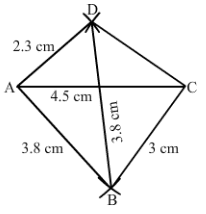Steps of construction:

Step I: Draw AC = 6 cm.

Step II: With A as the center and radius 3.8 cm, draw an arc.

Step III: With C as the center and radius 3.0 cm, draw an arc to intersect the arc drawn in Step II at B.

Step IV: With B as the center and radius 3.8 cm, draw an arc on the other side of AC.

Step V: With A as the center and radius 2.3 cm, draw an arc to intersect the arc drawn in Step IV at D.

Step VI: Join BA, DA, BC and CD to obtain the required quadrilateral.

Question: 2

Construct a quadrilateral ABCD in which BC = 7.5 cm, AC = AD = 6 cm, CD = 5 cm and BD = 10 cm.

Solution: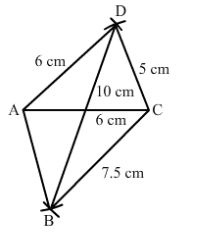Steps of construction:

Step I: Draw AC = 6 cm.

Step II: With A as the center and radius 6 cm, draw an arc.

Step III: With C as the center and radius 5 cm, draw an arc to intersect the arc drawn in Step II at D.

Step IV: With D as the center and radius 10 cm, draw an arc on the other side of the line segment AC.

Step V: With C as the center and radius 7.5 cm, draw an arc to intersect the arc drawn in Step IV at B.

Step VI: Join BA, DA, BC and CD to obtain the required quadrilateral.

Question: 3

Construct a quadrilateral ABCD, when AB = 3 cm, CD = 3 cm, DA = 7.5 cm, AC = 8 cm and BD = 4 cm.

Solution:

If we consider a triangle ABD from the given data, then

AB = 3 cm

BD = 4 cm

AB + BD = 3 + 4 = 7 cm

However, we know that the sum of the lengths of two sides of a triangle is always greater than the third side.

Therefore, construction is not possible from the given data.

Question: 4

Construct a quadrilateral ABCD given AD = 3.5 cm, BC = 2.5 cm, CD = 4.1 cm, AC = 7.3 cm and BD = 3.2 cm.

Solution: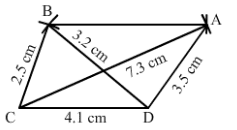Steps of construction:

Step I: Draw CD = 4.1 cm.

Step II: With C as the center and radius 7.3 cm, draw an arc.

Step III: With D as the center and radius 3.5 cm, draw an arc to intersect the arc drawn in Step II at A.

Step IV: With D as the center and radius 3.2 cm, draw an arc on the other side of AC.

Step V: With C as the center and radius 2.5 cm, draw an arc to intersect the arc drawn in Step IV at B.

Step VI: Join BA, DA, BC and BD and AC to obtain the required quadrilateral.

Question: 5

Construct a quadrilateral ABCD given AD = 5 cm, AB = 5.5 cm, BC = 2.5 cm, AC =7.1 cm and BD = 8 cm.

Solution: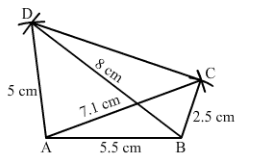Steps of construction:

Step I: Draw AB = 5.5 cm.

Step II: With A as the center and radius 7.1 cm, draw an arc.

Step III: With B as the center and radius 2.5 cm, draw an arc to intersect the arc drawn in Step II at C.

Step IV: With B as the center and radius 8 cm, draw an arc.

Step V: With A as the center and radius 5 cm, draw an arc to intersect the arc drawn in Step IV at D.

Step VI: Join DA, DB, BC, AC, and CD to obtain the required quadrilateral.

Question: 6

Construct a quadrilateral ABCD in which BC = 4 cm, CA = 5.6 cm, AD = 4.5 cm, CD = 5 cm and BD = 6.5 cm.

Solution: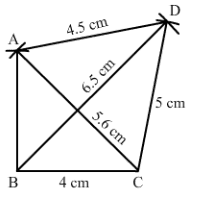Steps of construction:

Step I: Draw BC = 4 cm.

Step II: With B as the center and radius 6.5 cm, draw an arc.

Step III: With C as the center and radius 5 cm, draw an arc to intersect the arc drawn in Step II at D.

Step IV: With C as the center and radius 5.6 cm, draw an arc on the same side

Step V: With D as the center and radius 4.5 cm, draw an arc to intersect the arc drawn in Step IV at A.

Step VI: Join BA, AC, DA, BD, and CD to obtained the required quadrilateral.
```### Course Features

• 728 Video Lectures
• Revision Notes
• Previous Year Papers
• Mind Map
• Study Planner
• NCERT Solutions
• Discussion Forum
• Test paper with Video Solution